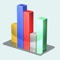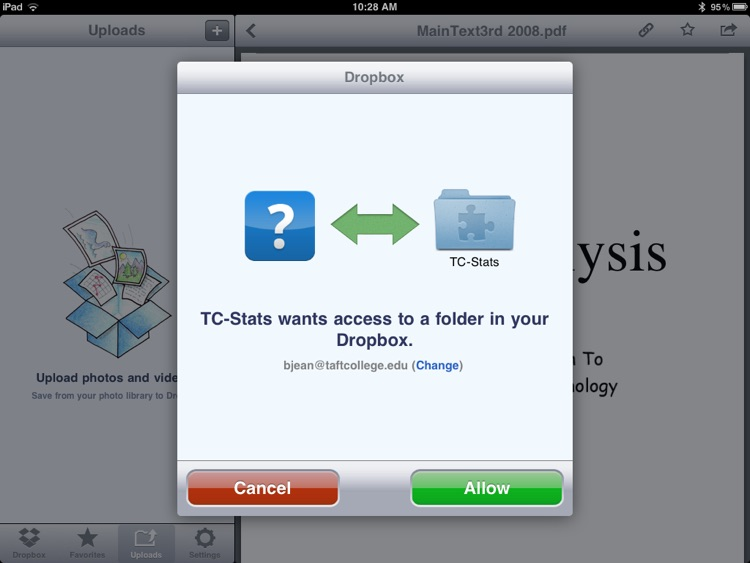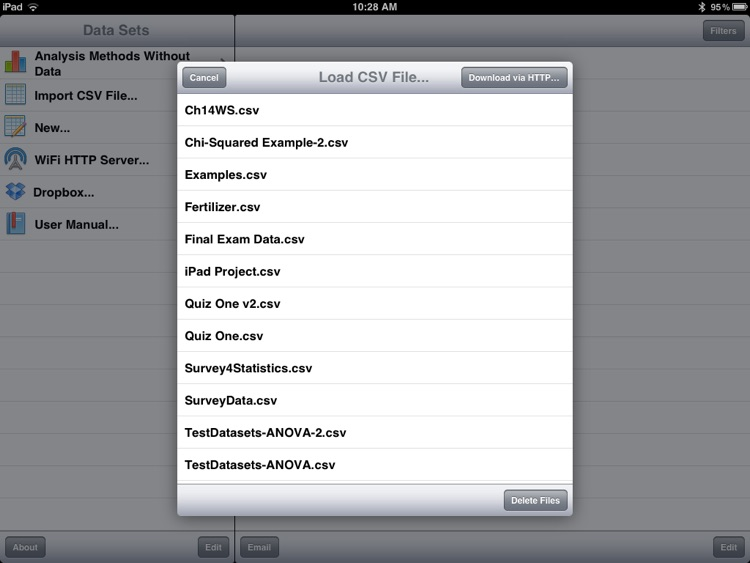## TC-Stats is a complete data analysis application for the iPad# TC-Stats

by Taft CollegeTC-Stats is a complete data analysis application for the iPad. The application performs the following functions: Summary Statistics, Histograms, Box-and-Whisker Plot, Normal Probability Plot, Scatter Plot, Frequency Distribution Table, Probability calculations based on the binomial, standard and non-standard normal, t, f and chi-squared distributions, one and two sample hypothesis tests based on the t and standard normal distributions, sign test, Wilkcoxon Rank-Sum, F-test, odds ratio, confidence intervals, one-way anova, Kruskal-Wallis test, chi-squared test of independence and goodness-of-fit, sample size calculations for inferences regarding a population mean or proportion.### App Details

Version
1.4.00
Rating
(2)
Size
151Mb
Genre
Education Utilities
Last updated
April 12, 2020
Release date
January 13, 2012

### App Screenshots### App Store Description

TC-Stats is a complete data analysis application for the iPad. The application performs the following functions: Summary Statistics, Histograms, Box-and-Whisker Plot, Normal Probability Plot, Scatter Plot, Frequency Distribution Table, Probability calculations based on the binomial, standard and non-standard normal, t, f and chi-squared distributions, one and two sample hypothesis tests based on the t and standard normal distributions, sign test, Wilkcoxon Rank-Sum, F-test, odds ratio, confidence intervals, one-way anova, Kruskal-Wallis test, chi-squared test of independence and goodness-of-fit, sample size calculations for inferences regarding a population mean or proportion.

TC-Stats imports data files in csv format and has Dropbox support and supports both stacked, and unstacked data for most techniques.